# Python Merge Sort Adjust the following code so that you can create random lists of numbers of lengths 10, 15, and 20. You will run the merge sort 10 times for each length. Record the run time for the...

Python Merge Sort

Adjust the following code so that you can create random lists of numbers of lengths 10, 15, and 20.

You will run the merge sort 10 times for each length. Record the run time for the length and then calculate the average time to sort.

Finally, compare the average run time for each length to the average time for the Merge Sort.

--------------------------------------------

Initial python code:

import random
import time

def mergeSort(alist):
print("Splitting ",alist)
if len(alist)>1:
mid = len(alist)//2
lefthalf = alist[:mid]
righthalf = alist[mid:]

mergeSort(lefthalf)
mergeSort(righthalf)

i=0
j=0
k=0
while i < len(lefthalf) and j < len(righthalf):
if lefthalf[i] < righthalf[j]:
alist[k]=lefthalf[i]
i=i+1
else:
alist[k]=righthalf[j]
j=j+1
k=k+1

while i < len(lefthalf):
alist[k]=lefthalf[i]
i=i+1
k=k+1

while j < len(righthalf):
alist[k]=righthalf[j]
j=j+1
k=k+1
print("Merging ",alist)

alist = [54,26,93,17,77,31,44,55,20]
mergeSort(alist)
print(alist)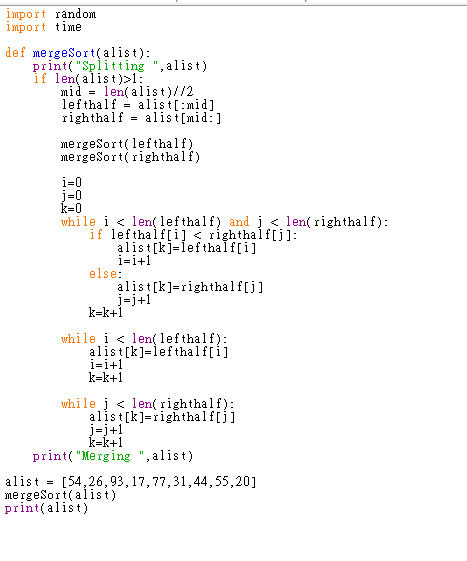import random mport tlme def mergeSort(alist) print"Splitting ",alist) if len(alist)>l: mid- len(alist)//2 lefthalf - alist:mid] righthalf - alist[mid: ] mergeSort(lefthalf) mergeSort( righthalf) i-0 While flefthal lefthal fighthajelen(righthalf) alist[kJ-leftha I f[i] i-i+1 else: alist[k]-righthalftj] j-j+1 k-k+1 while i

Hello, I have something to point out. The lengths 10, 15 or 20 is too low to calculate the run time for this merge sort. The results will always display 0 since the time required to sort lists of these lengths is very minute. To see any notable changes in time, we should use these values multiplied by 100 atleast. So in this program, I have used the lengths 1000, 1500 and 2000 instead of 10,15 and 20. You can change these values as you like, just modify the list called ‘lengths’ as you needed.

Here is the completed code for this problem. Comments are included, go through it, learn how things work and let me know if you have any doubts or if you need anything to change. If you are satisfied with the solution, please rate the answer. Thanks

#code

import random
import time

def mergeSort(alist):
if len(alist)>1:
mid = len(alist)//2
lefthalf = alist[:mid]
righthalf = alist[mid:]

mergeSort(lefthalf)
mergeSort(righthalf)

i=0
j=0
k=0
while i < len(lefthalf) and j < len(righthalf):
if lefthalf[i] < righthalf[j]:
alist[k]=lefthalf[i]
i=i+1
else:
alist[k]=righthalf[j]
j=j+1
k=k+1

while i < len(lefthalf):
alist[k]=lefthalf[i]
i=i+1
k=k+1

while j < len(righthalf):
alist[k]=righthalf[j]
j=j+1
k=k+1

#defining a list of lengths
lengths=[1000,1500,2000]
#an empty list to store average run times
avg_run_times=[]
#a variable to store total run time
total_run_time=0
#looping through each index in lengths list
for i in range(len(lengths)):
temp=0 #total run time for current length
#looping for 10 times

for k in range(10):
#creating a random list containing lengths[i] number of elements

aList=[random.randint(0,1000) for j in range(lengths[i])]
# recording start time

start = time.time()
#sorting

mergeSort(aList)
#recording end time

end = time.time()
#finding elapsed time in seconds

elapsed_time = end - start

temp+=elapsed_time
#finding average run time for current length

avg= temp/10

avg_run_times.append(avg)

total_run_time+=temp

#finding average time for performing sorting of all lengths
avg_time=total_run_time/(len(lengths)*10)
print('{:<15s} {:<15s} {:<15s} {:<15s}'.format('Length','Average Time', 'Final Average','Difference'))
#looping and printing length, average time, final average (average taken for all lengths),
#and the difference between average of current length and average as a whole

for i in range(len(lengths)):
print('{:<15d} {:<15.2f} {:<15.2f} {:<15.2f}'.format(lengths[i], avg_run_times[i], avg_time, avg_run_times[i]-avg_time))

#output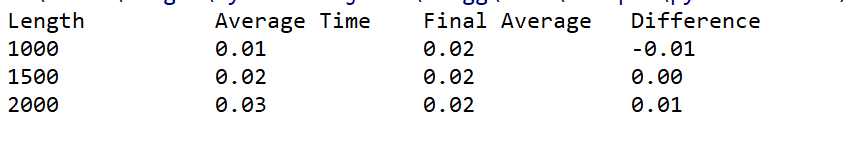##### Add Answer of: Python Merge Sort Adjust the following code so that you can create random lists of numbers of lengths 10, 15, and 20. You will run the merge sort 10 times for each length. Record the run time for the...
Similar Homework Help Questions
• ### In this assignment you will implement merge-sort algorithm by creating 2 threads instead of 2 pro...

In this assignment you will implement merge-sort algorithm by creating 2 threads instead of 2 processes. First half of the array will be sorted by thread 1 and the second half by thread 2. When the threads complete their tasks, the main program will merge the half arrays. You should not waste your time on merge-sort algorithm. Use the merge sort algorithm given below void mergesort(int a[],int i,int j) { int mid; if(i<j) { mid=(i+j)/2; mergesort(a,i,mid); //left recursion mergesort(a,mid+1,j); //right...

• ### Using Python, add an extra parameter k to the selection sort procedure. Modify the loop so that selection sort runs in l...

Using Python, add an extra parameter k to the selection sort procedure. Modify the loop so that selection sort runs in linear time for fixed k variable by returning early with only the first k items in the list sorted def selectionSortK(alist, k): for i in range(0,len(alist) - 1): min = i for j in range(i + 1, len(alist)): if i ==k: return; if alist[j]<alist[min]: min=j temp=alist[i] alist[i]=alist[min] alist[min]=temp

• ### Please give a output Linux/Ubuntu and how to run it (compile) this program ,my assigment is below...

Please give a output Linux/Ubuntu and how to run it (compile) this program ,my assigment is below : : Merge Sort algorithm using 2 processes a.) Define an integer array of 100 integers. Populate this array with random numbers. You can use int rand(void); function. Do not forget to initialize this function. You will sort the numbers in the array using merge-sort algorithm. In merge sort algorithm the half of the array will be sorted by one process and second...

• ### Here is my code for minesweeper in python and it has something wrong. Could you please help me to fix it? import tkinter as tk import random class Minesweeper: def __init__(self): self.main = tk.Tk()...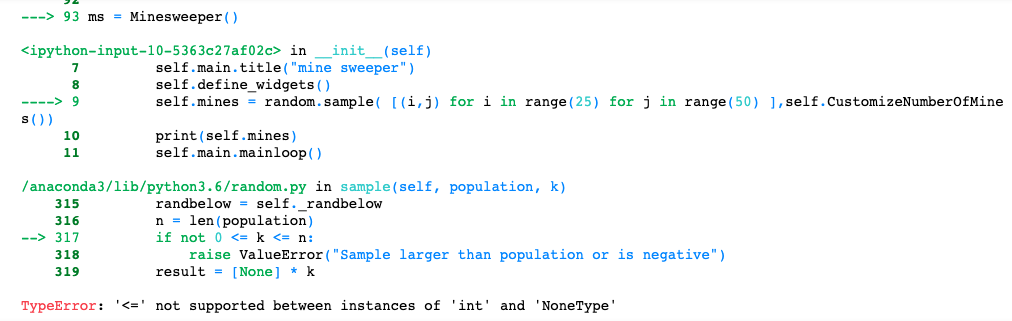Here is my code for minesweeper in python and it has something wrong. Could you please help me to fix it? import tkinter as tk import random class Minesweeper: def __init__(self): self.main = tk.Tk() self.main.title("mine sweeper") self.define_widgets() self.mines = random.sample( [(i,j) for i in range(25) for j in range(50) ],self.CustomizeNumberOfMines()) print(self.mines) self.main.mainloop() self.CustomizeNumberOfMines() def define_widgets(self): """ Define a canvas object, populate it with squares and possible texts """ self.canvas = tk.Canvas(self.main, width = 1002, height=502, bg="#f0f0f0") self.canvas.grid(row=0, column=0) self.boxes =...

• ### Modify the sorts (selection sort, insertion sort, bubble sort, quick sort, and merge sort) by adding code to each to tally the total number of comparisons and total execution time of each algorithm. E...

Modify the sorts (selection sort, insertion sort, bubble sort, quick sort, and merge sort) by adding code to each to tally the total number of comparisons and total execution time of each algorithm. Execute the sort algorithms against the same list, recording information for the total number of comparisons and total execution time for each algorithm. Try several different lists, including at least one that is already in sorted order. ---------------------------------------------------------------------------------------------------------------- /** * Sorting demonstrates sorting and searching on an...

• ### Your running times will probably be different than these. Please do a better job with the snipping tool than I did. Jav...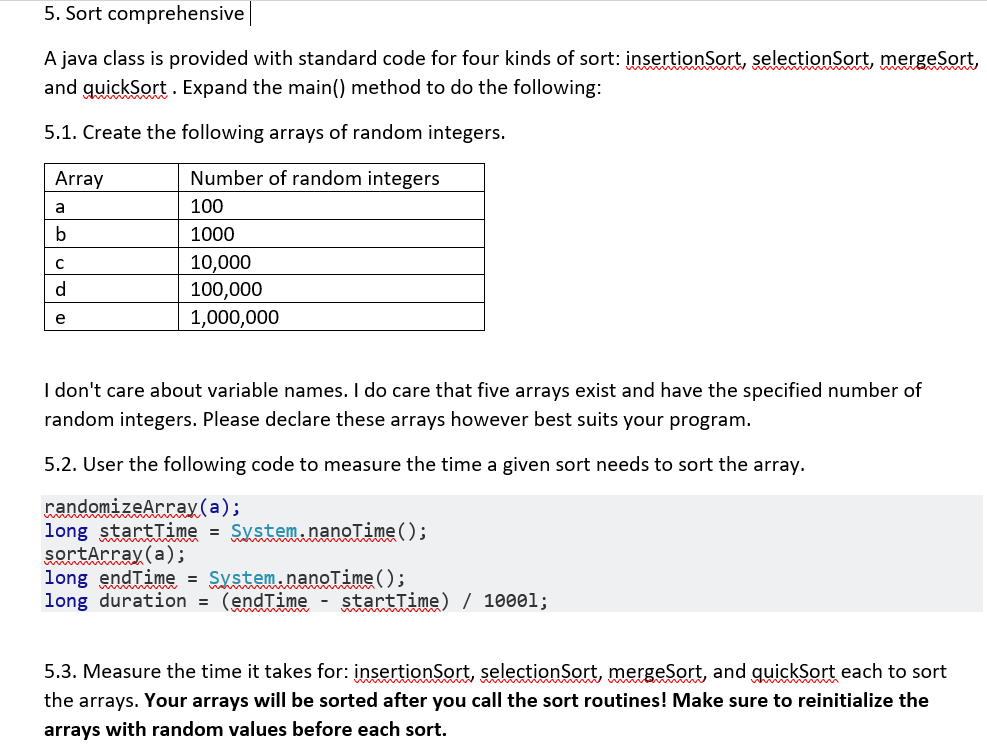Your running times will probably be different than these. Please do a better job with the snipping tool than I did. Java program provided: // Student Name Today's Date import java.util.Arrays; import java.util.Random; public class SortTimer {    // Please expand method main() to meet the lab requirements.       // You have the following sorting methods available:    // insertionSort(int[] a);    // selectionSort(int[] a);    // mergeSort(int[] a);    // quickSort(int[] a);    // The array will be in sorted order after the routines are called!   ...

• ### Practical 5: Write a program that implements several sorting algorithms, and use it to demonstrate the comparative perfo...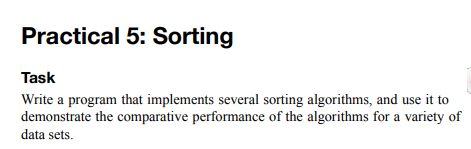Practical 5: Write a program that implements several sorting algorithms, and use it to demonstrate the comparative performance of the algorithms for a variety of data sets. Need Help With this Sorting Algorithm task for C++ Base Code for sorting.cpp is given. The header file is not included in this. Help would be much appreciated as I have not started on this due to personal reasons #include <cstdlib> #include <iostream> #include <getopt.h> using namespace std; long compares; // for counting...

• ### Any help in the compiler error //Driver Program //***************** /** * * CSCI 241 Assignment 8...

Any help in the compiler error //Driver Program //***************** /** * * CSCI 241 Assignment 8, Part 3 * * Author: your name * z-ID: your z-ID * Date: due date of assignment * * This program builds, sorts and prints lists using the quicksort and * merge sort algorithms. */ #include <iostream> #include <iomanip> #include <vector> #include <string> #include "sorts.h" #include "quicksort.h" #include "mergesort.h" using std::cout; using std::fixed; using std::left; using std::setprecision; using std::string; using std::vector; // Data files...

• ### c++ please read all question edit the program to test different random sizes of the array and give me the time in a file will be like random size of the array and next to it the time it took for each...

c++ please read all question edit the program to test different random sizes of the array and give me the time in a file will be like random size of the array and next to it the time it took for each size Im trying to do time analysis for Quick sort but i keep getting time = 0 also i want edit the program to test different random sizes of the array and give me the time in a...

• ### Need code for 1 through 8 please. Code below was given for the problem # Copyright 2017, 2013,...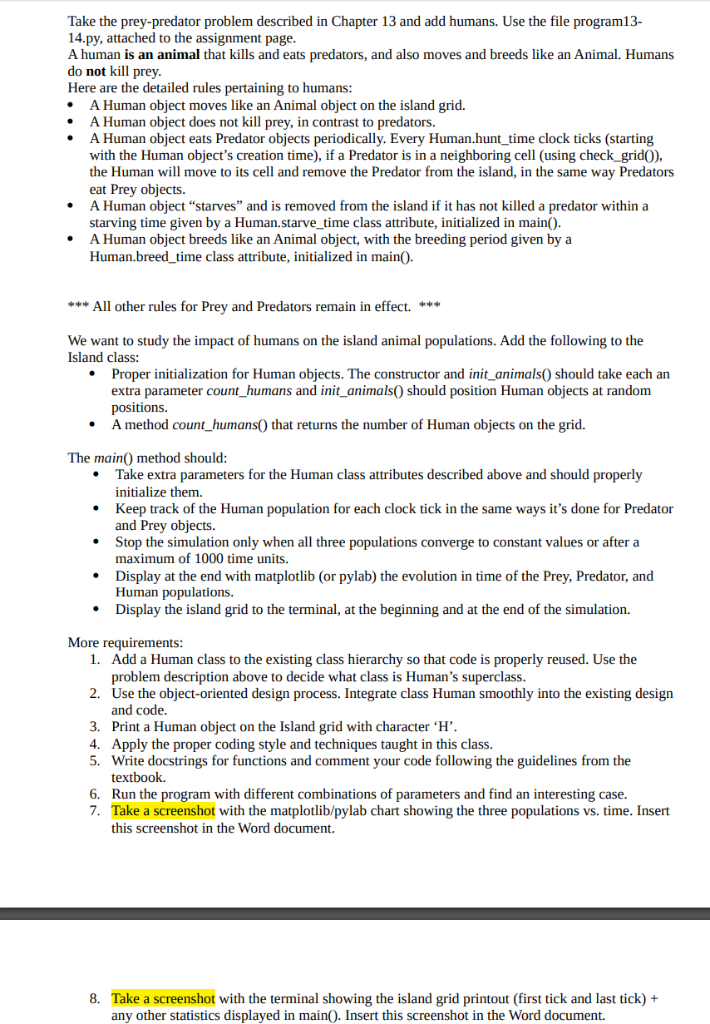Need code for 1 through 8 please. Code below was given for the problem # Copyright 2017, 2013, 2011 Pearson Education, Inc., W.F. Punch & R.J.Enbody """Predator-Prey Simulation four classes are defined: animal, predator, prey, and island where island is where the simulation is taking place, i.e. where the predator and prey interact (live). A list of predators and prey are instantiated, and then their breeding, eating, and dying are simulted. """ import random import time import pylab class Island...

Need Online Homework Help?